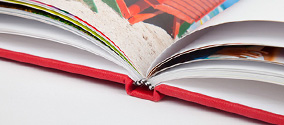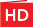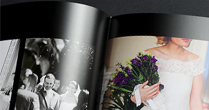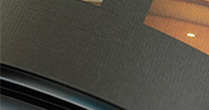# Photo Books Pricing

There’s a book for every budget - from Softcover books to Premium Material covers. Use our pricing calculator below to choose the Photo Book that is going to bring your memories to life or have a play with all the options to find the right product for you.

### Select Binding### Choose Paper / Quality

SD

#### Standard Definition#### High Definition### Select Product Size

6 x 6" Calculating Calculating Calculating
8 x 6" Calculating Calculating Calculating
8 x 8" Calculating Calculating Calculating
8 x 11" Calculating Calculating Calculating
11 x 8.5" Calculating Calculating Calculating
12 x 12" Calculating Calculating Calculating
16 x 12 " Calculating Calculating Calculating

Page Count:
Calculating
Included Free: Included:
Calc
Minimum:
Calc
Maximum:
Calc

Calculating

Calculating

Calculating

Calculating

Calculating